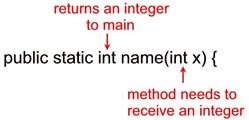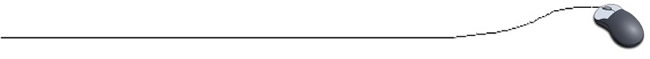Methods -- Style 4
(does receive arguments, does return values)Style 4: Our fourth style of method takes arguments AND returns a value.

//Demo Program for Methods

public class method4test {
public static void main(String[ ] args)  {

int test1 = 12, test2 = 10;
// notice in this next line the method named "min" is in a print statement
System.out.println("The minimum of " + test1 + " and " + test2
+ " is " + min(test1,test2) + ".");
}

//Method to find minimum
// This method needs to receive two integers and returns one integer.

// Notice the names of the variables sent (test1, test2),
// and the parameters receiving them (int x, int y) are not the same.

public static int min(int x, int y)
{
int minimum;  // local variable
if (x < y)
minimum = x;
else
minimum = y;
return (minimum);  // return value to main
}
}

Output:

 The minimum of 12 and 10 is 10.